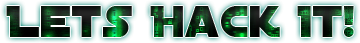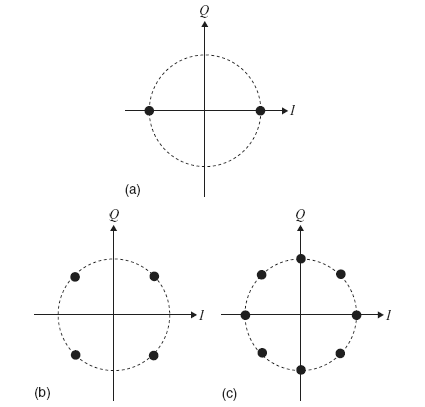# Binary Phase Shift Keying Modulation

First things first. We successfully acquired a LRIT signal from GOES. Our first step is to demodulate the baseband signal from a SDR like Airspy or RTLSDR and transform this to a symbol stream. For the easy of use, I will use GNU Radio for assembling the demodulator.

According to the spec the LRIT signal is a BPSK (Binary Phase Shift Keying) modulated that has a symbol rate of 293883 symbols / second. The first thing is: How a BPSK Modulation works?

First we have two signals: The Carrier Wave and the Binary Stream that we want to send. The Carrier Wave is a Sine signal that will carry the modulated data along the path. It’s frequency is usually way higher than the bitrate or symbol rate. In BPSK Modulation what we do is basically  change the phase of the signal. Since its a **binary **phase shift, we basically only invert the phase of the signal, and that is basically invert the local polarity of the wave. You can see in the picture below that when we change the polarity we generate “notches” over the carrier wave. That’s our bits being modulated into the carrier wave using a binary phase shift. The advantage about changing phase is that we only need to have a consistent phase in the receptor, not necessarily a very huge amplitude of the signal.Basically what we have is:

• When our bit is 1, our carrier wave phase shift is 0 degrees.
• When our bit is 0, our carrier wave phase shift is 180 degrees (inverted)

Simple isn’t it? So we can represente also our data in a phase-diagram by having two unitary vectors I and Q. Below there are some representations of Phase Diagram for BPSK (a), QPSK (b) and 8-PSK (c).So for BPSK in our phase diagram we basically have: for Positive I (quadrants 0 and 3) we have bit 0, for Negative I ( quadrants 1 and 2) we have bit 1.

Just for reference, in QPSK (b) we would have a gray coded binary values:

1. Quadrant we would have 00
2. Quadrant we would have 01
3. Quadrant we would have 11
4. Quadrant we would have 10

Same works for 8-PSK, but with 3 bits instead of 2. Usually the binary code is gray-coded starting from 0 and increasing counter-clockwise.